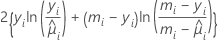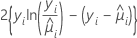# Methods and formulas for the analysis of variance in Binary Fitted Line Plot

Select the method or formula of your choice.

## Analysis of variance

Deviance measures the discrepancy between the current model and the full model. The full model is the model that has n parameters, one parameter per observation. The full model maximizes the log-likelihood function. The full model provides a point of comparison for models with fewer than n parameters. Comparisons to the full model use the scaled deviance.

The contribution to the scaled deviance from each individual data point depends on the model.

Model Deviance
BinomialPoissonThe deviance table is constructed based on the following general result which assumes that ϕ is known. If DI is the deviance associated with an initial model and DS is the deviance associated with a subset of terms in the initial model, then under some regularity conditions, the following relationship exists:

The difference between the deviances is asymptotically distributed as a chi-square distribution with d degrees of freedom. These statistics are calculated for adjusted (type III) analysis and sequential (type I) analysis. The adjusted deviance and the chi-square statistic in the deviance table are equal. The adjusted mean deviance is the adjusted deviance divided by the degrees of freedom.

For the sequential analysis, the output depends on the order that the predictors enter the model. The sequential deviance is the unique portion of the deviance that a predictor explains, given any predictors already in the model. If you have a model with three predictors, X1, X2, and X3, the sequential deviance for X3 shows how much of the remaining deviance that X3 explains given that X1 and X2 are already in the model. To obtain a different sequential deviance, repeat the regression procedure entering the predictors in a different order.

If ϕ is unknown, as for responses that follow a normal distribution, then under some regularity conditions the relationship changes to the following:

Here, the difference between the deviances is asymptotically distributed as an F distribution with d degrees of freedom for the numerator and np degrees of freedom for the denominator. To estimate the dispersion parameter, use the initial model.

### Notation

TermDescription
yithe number of events for the ith rowthe estimated mean response of the ith row
mithe number of trials for the ith row
Lfthe log-likelihood of the full model
Lcthe log likelihood of the model with a subset of terms from the full model
dthe degrees of freedom are the difference between the numbers of parameters in the models to compare
ϕthe dispersion parameter, known to be 1 for the binomial and the Poisson models
nthe number of rows in the data
pthe regression degrees of freedom for the initial model

## Degrees of freedom (DF)

Indicates the number of independent pieces of information involving the response data needed to calculate the adjusted mean deviances. The degrees of freedom for each component of the model are:
 Source of variation DF Regression p Error n − p − 1 Total n − 1 Continuous predictors 1 Categorical predictors q − 1

### Notation

TermDescription
pThe sum of the degrees of freedom for the predictors. The predictors do not include the constant.
nThe number of observations in the data set
qThe number of levels of the categorical predictor

## Log-likelihood

The log-likelihood functions are parameterized in terms of the means. The general form of the functions follow:

The general form of the individual contributions follows:

The specific form of the individual contributions depends on the model.

 Model li BinomialPoisson### Notation

TermDescription
yithe number of events for the ith row
mithe number of trials for the ith rowthe estimated mean response of the ith row

## p-value (P)

Used in hypothesis tests to help you decide whether to reject or fail to reject a null hypothesis. The p-value is the probability of obtaining a test statistic that is at least as extreme as the actual calculated value, if the null hypothesis is true. A commonly used cut-off value for the p-value is 0.05. For example, if the calculated p-value of a test statistic is less than 0.05, you reject the null hypothesis.

By using this site you agree to the use of cookies for analytics and personalized content.  Read our policy